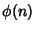## Leudesdorf Theorem

Letdenote the set of thenumbers less than and Relatively Prime to, whereis the Totient Function. Then ifthenHardy, G. H. and Wright, E. M. A Theorem of Leudesdorf.'' §8.7 in An Introduction to the Theory of Numbers, 5th ed. Oxford, England: Clarendon Press, pp. 100-102, 1979.